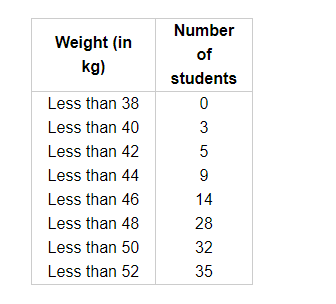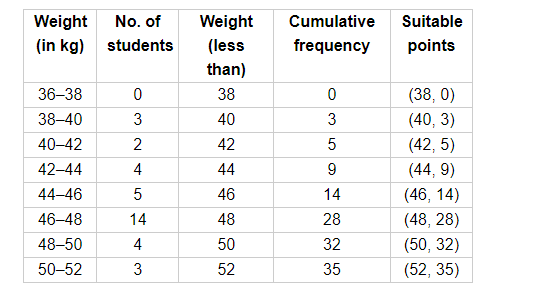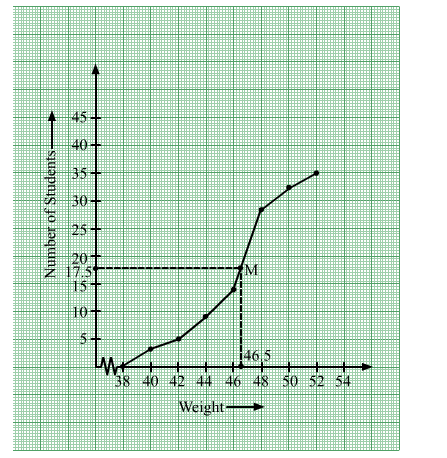# During the medical check up of 35 students of a class,

Question:

During the medical check up of 35 students of a class, their weights were recorded as follows:Draw a less than type ogive for the given data. Hence, obtain the median weight from the graph and verify the result by using the formula.

Solution:

Prepare the table for cumulative frequency for less than type.Now, draw the less than ogive using suitable points.Here, $\frac{N}{2}=\frac{\sum f}{2}==\frac{35}{2}=17.5$

From (0, 17.5) draw a line parallel to horizontal axis, which intersects the graph at the point M having x-coordinate as 46.5.

Therefore, the median is 46.5 kg.

Calculation for median using formula.

Now, $\frac{N}{2}=\frac{35}{2}=17.5$

So, 46–48 is the median class.

Here, $l=46, h=2, f=14, F=14$

Median $=l+\frac{\frac{N}{2}-f}{f} \times h$

$=46+\frac{\frac{35}{2}-14}{14} \times 2$

$=46+0.5=46.5 \mathrm{~kg}$

Hence, both the methods give same result.